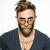# Can You Score 10/15 In This Impossible Math Drill?

## Try this very difficult math drill to find out if you really are the best of the best. Take a deep breath and let's start.Created by Terry Stein
On Aug 30, 2021

Do you sometimes feel misunderstood? Do you have a thing for equations? Maybe you have the traits of geniuses or maybe you truly are a genius!

1 / 15

25 x 25 = ?

2 / 15

4 + (8 – 4) 4 = ?

3 / 15

6 ÷ 2 (2 + 1) = ?

4 / 15

When you add the square root of 100 to 100 squared, what do you get?

5 / 15

Which is divisible by 7 without a remainder?

6 / 15

Pick the dimensions of a square whose perimeter and area are EQUAL in value?

7 / 15

Pick 3 different numbers whose product and sum always give the same answer.

8 / 15

What is the square root of 4,096?

9 / 15

1 + 1 ÷ 1 + 1 x 1 = ?

10 / 15

3 − 3 + 3 × 3 = ?

11 / 15

Convert five percent to decimals.

12 / 15

9 ÷ 3 + 4 (11 − 5) = ?

13 / 15

If X is bigger than Y but smaller than Z, then:

14 / 15

4 + 2 (8 – 6) = ?

15 / 15

My grandson weighs 44 pounds plus half his own weight. How much does he weigh?

15
Questions left

### So how much did you like this quiz?

Calculating results